# Flat galaxy rotation curves without dark matter

I need someone with more experience in the field who has knowledge of excel to check over my work.

Given the gravitational attraction between two bodies is F = (GMm)/r^2, and the centripetal force required to keep a body in orbit is F = (mv^2)/r, therefore

(mv^2)/r = (GMm)/r^2 therefore M=(v^2*r)/G

Therefore for any given star in the galaxy we can determine how much force is needed to keep it in orbit if we know its distance from the galactic core and its rotational velocity. And we can estimate the mass within the orbit of that body which is holding it in orbit.

But F = (GMm)/r^2 describes the force between two bodies, such as the Earth and the Sun. But the galaxy does not resemble a solar system with one dominating mass with several orbiting bodies, but rather an interconnected mesh of stars, all equally massive, and all pulling on each other. Therefore using F = (GMm)/r^2 has lead to incorrect calculations about how much mass is needed to produce the flat rotational curve that we observe due to the inverse square law of gravity. This leads to an overestimation of the amount of mass needed in the galaxy to keep a star in orbit.

To explore this possibility I made an excel spreadsheet that uses several of the known characteristics of the milky way (flat rotation of 225km/s, ~100 billion solar masses, diameter of galactic bulge, diameter of the disk, etc) and constants (avg solar mass, c, etc) and makes a grid that represents the Milky Way and populates each grid based on normal probability distribution of stars (Actually two overlapping normal distributions, one for the bulge and one for the disk).

Then it can calculate the distance from a star of interest, to the rest of the points on the grid and estimate the gravitational attraction for each of the grid points.

With a distribution of matter in the galaxy that looks like this: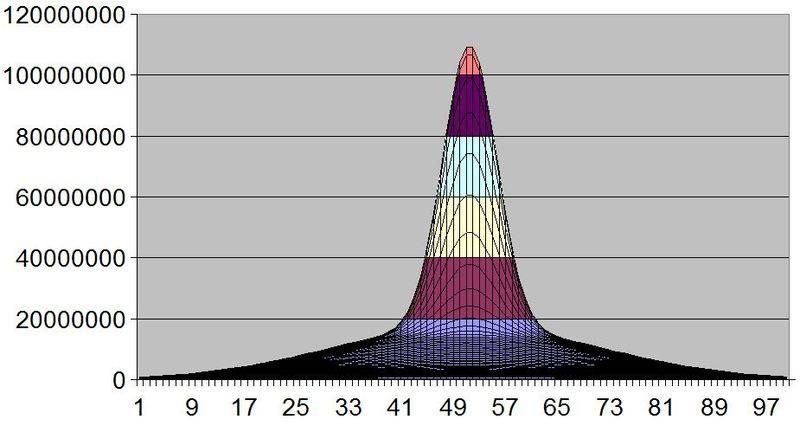And with a flat galaxy rotation curve that looks like this: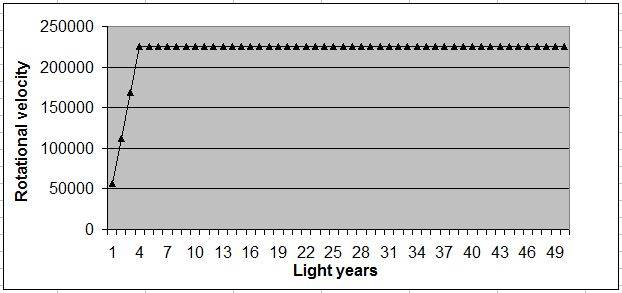I can get a pretty good balance of the forces required to keep a star in orbit, vs the amount of force that I can compute from the distribution of stars.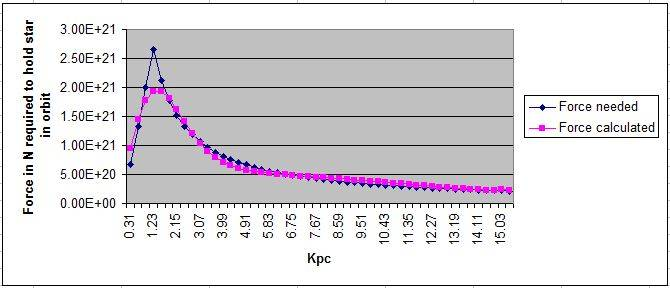For example, our sun is at a distance of 27000 light years from the galactic core, and here is the gravity profile that I came up with. The spike below is the gravity from local stars pulling us out of orbit, and the spike above is the gravity from local stars pulling us towards the galactic core, and there is just a faint hint at where the galaxy center is. 80% of the gravitational contribution keeping us in orbit is is coming from the local disk stars, with only 20% coming from the galactic bulge. And a significant portion of stars are actually working to pull us away from the galactic core. But there is enough mass inside our orbit to overcome it.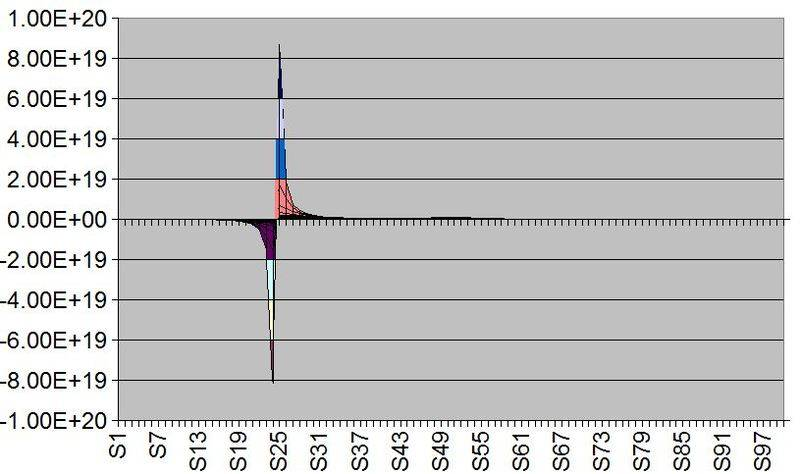At 50000 light years from the galactic core, 90% of the gravity holding the star in orbit is coming from local disk stars, and only 10% from the galactic bulge.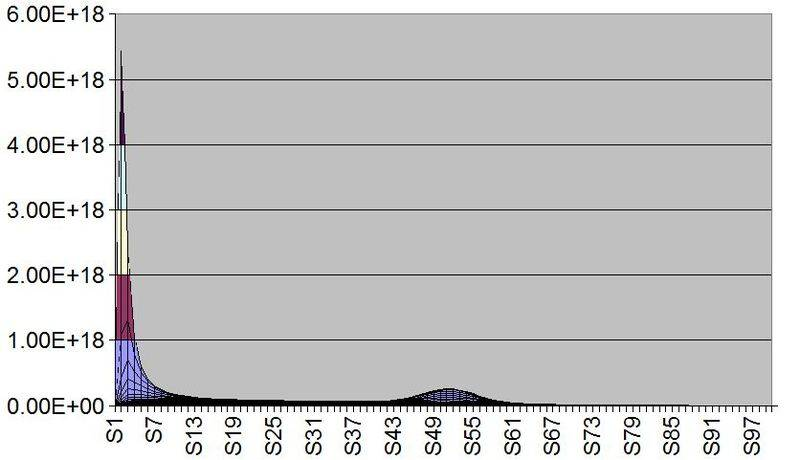In this way the inverse square law works in our favor and reduces the amount of mass needed inside the orbit to keep it gravitationally bound. The effect gets stronger as you get further from the galactic core. So that at the very edge of the galaxy M=(v^2*r)/G overstates the amount of mass needed to keep a star in orbit by 70%, and dark matter was invented to make up for this supposed shortfall in mass. But I think that I have shown that you do not need to invoke dark matter to explain the flat galactic rotation curve.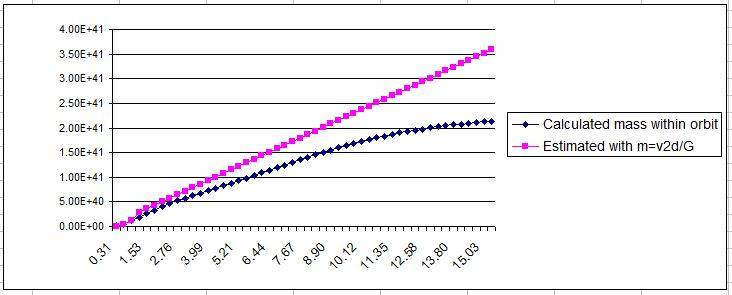Look over the spreadsheet and tell me what you think.

http://mike-stalkfleet.com/images/Gravity_in_Milky_Way_ver3.xls

Drakkith
Staff Emeritus